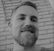# App Development

Announcements
See why Qlik was recognized for the seventh year in a row – and discover how we can help you tackle your data integration challenges. Get the report
cancel
Showing results for
Did you mean:Creator

## GRAPH Year over Year Growth per Product Category

Hi,

I was able to get the year over last year growth using the FUNCTION above, and that worked properly in a Graph, that only have DIMENSION year and the Following Measure

sum(

{<

[YEAR_INVOICE]={">2010"}-{"\$(=Max([YEAR_INVOICE]))"}

>}

[SALES])

/

Above(sum(

{<

[YEAR_INVOICE]={">2010"}-{"\$(=Max([YEAR_INVOICE]))"}

>}

[SALES]))

-1

BUT when I ad a new dimension in the GRAPH, to split the information in Product_Categories, then does not work. I guess this formula using ABOVE does not make sense then.

¿Any suggestions?

1 Solution

Accepted SolutionsMVP

Try this

Aggr(

Sum({<[YEAR_INVOICE]={">2010"}-{"\$(=Max([YEAR_INVOICE]))"}>} [SALES])

/

Above(Sum({<[YEAR_INVOICE]={">2010"}-{"\$(=Max([YEAR_INVOICE]))"}>}[SALES]))

-1,

Product_Categories, YEAR_INVOICE)

This may still not work if YEAR_INVOICE is not sorted in ascending order in the script... but this could be handled if you are using QV12 or above or Qlik Sense (The sortable Aggr function is finally here!)

Aggr(

Sum({<[YEAR_INVOICE]={">2010"}-{"\$(=Max([YEAR_INVOICE]))"}>} [SALES])

/

Above(Sum({<[YEAR_INVOICE]={">2010"}-{"\$(=Max([YEAR_INVOICE]))"}>}[SALES]))

-1,

Product_Categories, (YEAR_INVOICE, (NUMERIC)))

2 RepliesMVP

Try this

Aggr(

Sum({<[YEAR_INVOICE]={">2010"}-{"\$(=Max([YEAR_INVOICE]))"}>} [SALES])

/

Above(Sum({<[YEAR_INVOICE]={">2010"}-{"\$(=Max([YEAR_INVOICE]))"}>}[SALES]))

-1,

Product_Categories, YEAR_INVOICE)

This may still not work if YEAR_INVOICE is not sorted in ascending order in the script... but this could be handled if you are using QV12 or above or Qlik Sense (The sortable Aggr function is finally here!)

Aggr(

Sum({<[YEAR_INVOICE]={">2010"}-{"\$(=Max([YEAR_INVOICE]))"}>} [SALES])

/

Above(Sum({<[YEAR_INVOICE]={">2010"}-{"\$(=Max([YEAR_INVOICE]))"}>}[SALES]))

-1,

Product_Categories, (YEAR_INVOICE, (NUMERIC)))Creator
Author

Thanks You stalwar1